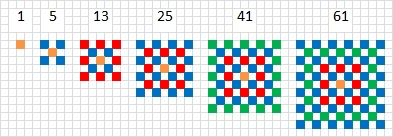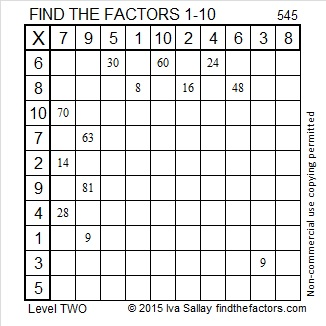# 545 and Level 2

The first few centered square numbers are 1, 5, 13, 25, 41, and 61. Starting in the center of this multi-colored square, can you locate each of those centered square numbers? 545 is the 17th centered square number. This wikipedia link explains the relationship between centered square numbers and the more familiar square numbers like 1, 4, 9, 16, 25 and 36. It also explains that every centered square number except 1 is the hypotenuse of a Pythagorean triple.If you have difficulty seeing those first few centered square numbers, perhaps this will help:545 is a centered square number because 16 and 17 are consecutive numbers and (16^2) + (17^2) = 545.

It is probably less exciting that (23^2) + (4^2) = 545.

545 is the hypotenuse of four Pythagorean triples. Which of these triples are primitives and which of them aren’t? The ones with greatest common factors greater than one are not primitive:

• 33-544-545
• 184-513-545
• 300-455-545
• 327-436-545Print the puzzles or type the solution on this excel file: 10 Factors 2015-07-06

—————————————————————————————————

• 545 is a composite number.
• Prime factorization: 545 = 5 x 109
• The exponents in the prime factorization are 1 and 1. Adding one to each and multiplying we get (1 + 1)(1 + 1) = 2 x 2 = 4. Therefore 545 has exactly 4 factors.
• Factors of 545: 1, 5, 109, 545
• Factor pairs: 545 = 1 x 545 or 5 x 109
• 545 has no square factors that allow its square root to be simplified. √545 ≈ 23.345235—————————————————————————————————## 3 thoughts on “545 and Level 2”

1.Thumbup

No?

•ivasallay

I’ve updated the post. I hope that helps. (If it doesn’t, help me out with my attempt to read your mind.)

•Thumbup

ivasallay,
It’s not you, it’s me.
I am not too smart in this area.
Thank you for the effort of updating it.

This site uses Akismet to reduce spam. Learn how your comment data is processed.